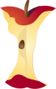Home > Grade Levels > Grade 8 >

## Graphing Proportional Relationships#### Aligned To Common Core Standard:

Grade 8 Number System- 8.EE.B.5

### Printable Worksheets And Lessons

• Faster Paces Step-by-Step Lesson- Two boys are riding bikes. Which one moves at a faster pace?

• Guided Lesson - All the problems compare a graph to an equation. So kids find it easier to graph the equation. Yet others find it easier to determine the equation of the graph.

• Guided Lesson Explanation - I like equations and for the answers I put everything into an equation. I will come back and work on this one to have a graph comparison version later this month.

• Independent Practice - It's a big battle royale of graphs versus equations.

• Matching Worksheet -This one is pretty easy because it's matching. I would insist on everyone showing their work.

###Answer Keys

View Answer Keys- All the answer keys in one file.

###(Click Here to Upgrade)

#### Homework Sheets

Compare the graph and the equation without any change.

#### Practice Worksheets

The color of the line dot here was requested by many teachers. It shows up well on Smart boards.

#### Math Skill Quizzes

Because of the size of the graph, I can only get two questions on each page.

 Quiz 1 Quiz 2 Quiz 3